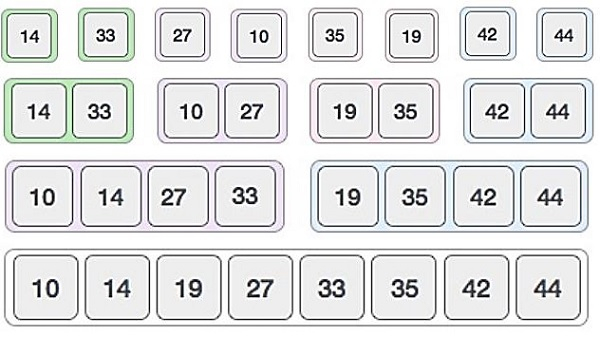# Merge Algorithms in Data Structure

The Merge algorithm is used to merge two sorted list into one list. This algorithm is used in different cases. If we want to perform merge sort, then we need to merge the sorter lists into larger lists.

The approach is simple. We take two lists, there will be two pointers. The first one will point to the element of the fist list, second one will point to the elements of the second list. Based on their values, the smaller element is taken from one of these two lists, then increase the pointer of that corresponding list. This operation will be performed until one list is exhausted. After that add the remaining list at the end of the final merged list.

Let us see the illustration to get better idea.## Algorithm

Merge(array, left, middle, right) −

Begin
nLeft := m - left+1
nRight := right – m
define arrays leftArr and rightArr of size nLeft and nRight respectively
for i := 0 to nLeft do
leftArr[i] := array[left +1]
done
for j := 0 to nRight do
rightArr[j] := array[middle + j +1]
done
i := 0, j := 0, k := left
while i < nLeft AND j < nRight do
if leftArr[i] <= rightArr[j] then
array[k] = leftArr[i]
i := i+1
else
array[k] = rightArr[j]
j := j+1
k := k+1
done
while i < nLeft do
array[k] := leftArr[i]
i := i+1
k := k+1
done
while j < nRight do
array[k] := rightArr[j]
j := j+1
k := k+1
done
End

Updated on: 11-Aug-2020

497 Views